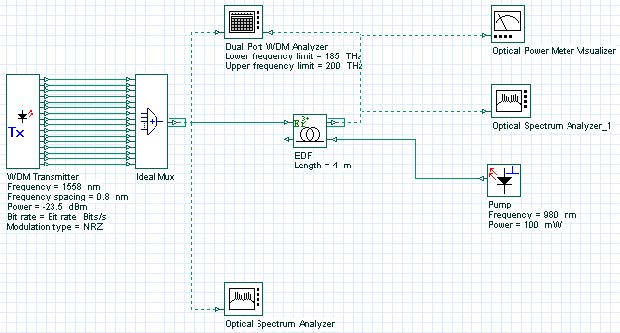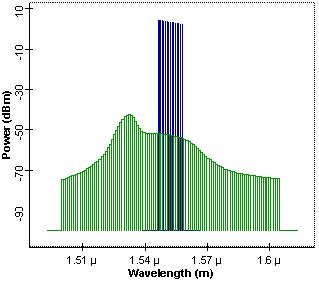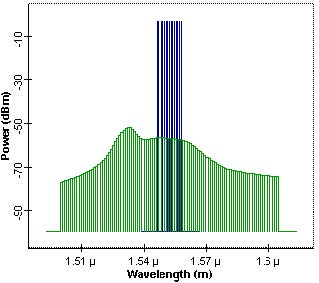In this tutorial, we provide an example of the gain flatness optimization type. The gain of the EDFA will be flattened by optimizing the fiber length and pump power.
One difficulty in implementing a WDM system including EDFA’s is that the EDFA gain spectrum is wavelength dependent. This effect results in SNR differential between channels after passing through a cascade of EDFA’s. Several method to correct this gain non-uniformity are suggested, for example using internal  or external filters , or thermally decreasing the homogeneous line broadening of the amplifier . These methods require either extra components or are complicated. A promising method is suggested in . This method is based on optimizing the EDFA itself by controlling the fiber length and pump power for a given input power and desired output power.
In this example we show how to flatten the gain of an EDFA by using this technique. The project layout is shown in Figure 1.Figure 1: Project layout of gain optimized EDFA

Optimization targets are chosen as in  to have a comparison. However, note that the fiber parameters in our simulation might be different than that of  since no value is given there. The input of EDFA is 16 equalized wavelength multiplexed signals in the wavelength region of 12 nm (1546-1558 nm) with 0.8 nm separations. Power of each channel is -26 dBm. We have used default fiber parameters. The desired gain is 23 dB. We also want an output power of more than 8.5 dBm and a gain flatness (defined as Gmax/Gmin) of less than 0.5 dB.

Fiber length and pump power is selected as parameters to be optimized to achieve the desired gain under output power and gain flatness constraints. The Dual Port WDM Analyzer measures gain and flatness, whereas the Optical Power Meter measures the output power. Initial parameter values are as follows: Pump power is 100 mW; fiber length is 4 m. The pump power is bound between 0 and 160 mW. The fiber length is bound between 1 and 40 m. Parameter termination tolerance is 1, result and constraints termination tolerance is 0.1.

Note that no unit for parameters and results is indicated in the optimization tool. The units of parameters and results in optimization tool are taken to be same as the ones that are given in project layout for the corresponding parameter or result.Figure 2: Signal and noise spectrum of an un-optimized EDFA

Figure 2 shows an un-optimized amplifier output signal and noise spectrum when pump power and fiber length are 100 mW and 4 m. In this case, even though the average gain is about 30 dB, gain flatness is about 2.24 dB, which is much higher than required.Figure 3: Output signal and noise power spectrum

After 24 passes, pump power and fiber length are optimized to achieve the desired goals. Optimum pump power and fiber length are found to be 24.13 mW and approximately 5.22 m.

At these values, an average gain of 23 dB and a gain flatness of 0.29 dB are achieved. The output signal power is about 8 mW. The output signal and noise spectrum is shown in Figure 3.

These results are comparable to results of  where optimization is done by using a semi-analytical method and then the findings are confirmed experimentally. Compared with the results of , our simulation results in a better gain flatness.

References:
M. Tachibana, et al., “Erbium-Doped Fiber Amplifier with Flattened Gain Spectrum”, IEEE Photon. Tech. Lett. 3, 118 (1991).

S. F. Su, et al., ” Flattening of Erbium-doped fiber amplifier gain spectrum using an acousto-optic tunable filter”, Electron. Lett. 29, 477 (1993).

E. L. Goldstein, et al., “Inhomogeneously broadened fiber amplifier cascade for transparent multiwavelength lightwave networks”, J. Light. Tech. 13, 782 (1995).

M. A. Ali, et al., “Performance of erbium-doped fiber amplifier cascades in WDM multiple access lightwave networks”, IEEE Photon. Tech. Lett. 6, 1142 (1994).

S. Y. Park, et al. “Doped fiber length and pump power of gain-flattened EDFAs”, Elect. Lett. 32, 2161 (1996).